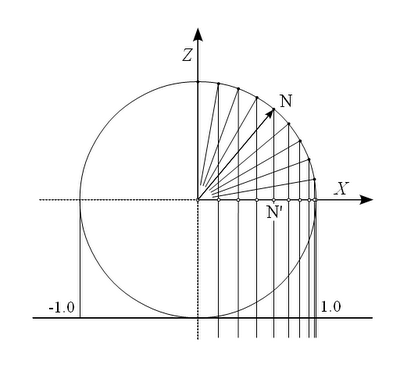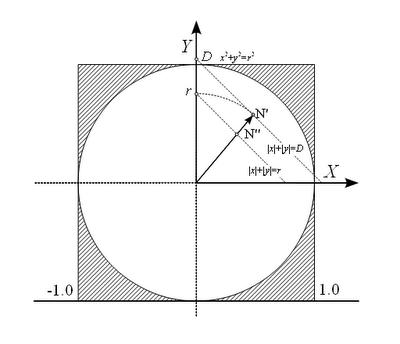## Monday, July 18, 2011

### Encoding normals for Gbuffer

Deferred shading has nowadays become nearly ubiquitous in real-time rendering due to the separation of geometry and lighting passes. The general idea of this technique is to render all important properties (color, normal, reflectivity and so on) of all visible surfaces to a set of screen-sized textures. Later rendering passes (lighting) get surface parameters from those textures and thus complex geometries do not have to be rendered multiple times.

One design problem of Gbuffer implementation is the efficient encoding of surface normals. Normals in three-dimensional space have three components:

N = Nx,Ny,Nz

The most intuitive way would be to store those in three texture channels. But this would be wasteful, because we know, that:

Nx2 + Ny2 + Nz2 = 1

Gbuffer requires many texture channels for other data, thus we want to cut off as many unnecessary channels as possible to conserve memory and fillrate.

Another simple alternative would be to store only two components (Nx and Ny) and reconstruct third (Nz) in pixel shader. Unfortunately we will lose the sign of Nz in that process. While in ideal scene there should be no normals with negative Z (facing away from camera), they happen in real scenes because of realistic polygon counts.

There exist several techniques for encoding normals while (at least partially) preserving Z component. Unfortunately no one of those is ideal. Either they are not able to encode full range of Z (various projections) or waste precision and calculation (polar coordinates).

While implementing foliage shader for Khayyam I needed a way to encode full range of possible normals into two-component vector (that will later be stored in 4-component 8-bit RGBA texture of Gbuffer). The following is the brief introduction of the technique used. It solves all the problems I am aware of - with the price of slightly more shader instructions than simple projection encoders.

Efficient projection of normal space

We forget for awhile the need of storing the sign of Z component and look, how we can store X and Y components most efficently.
The trivial way is to simply forget Z and store X and Y. In geometric terms it means projecting the hemisphere of normal space to an unit circle on XY plane along Z vector as in following figure:The trivial projection of normal space to XY plane

The problem with this projection is, that the uniform angular difference between normals near equator gives much smaller difference in X and Y coordinates than near poles. If the precision of normal texture is limited to 16 bits (4x8 bit or 2x16 bit integer buffers) or even less (FP16 buffers) , the loss of precision may become visible. For example, for 16 bit buffers, the minimum representable angle near equator is:

acos (1 - 2e-15) = 1.44°

Near poles it is much better:

asin (2e-15) = 0.002°

Clearly we are wasting precision near poles and introduce errors near equator.

To get better distribution of precision, we can switch projection from parallel to radial, with the projection center at the point (0,0,-1) as on the following figure:The radial projection of normal space

The distribution of angles is still not uniform but is clearly much better (it differs only by the factor of 2 at poles and equator).
Actually, having the center of projection at (0,0,-sqrt(2)) would result in even better distribution, but I wanted to keep it at (0,0,-1) to simplify calculations a bit.

The hemisphere is still projected at unit circle at XY plane, although now we cannot project the normals on negative hemisphere directly but have to take the absolute value of Z coordinate before projecting.

This solves the problem of effecient use of normal texture precision. Now we can go on to encoding the sign of Z into the same buffer.

Encoding the sign of normal Z coordinate

Our positive hemisphere of normal space got projected to unit circle on XY plane as in following figure:The normal space projection to XY plane and compaction schema

As we can see, we are actually wasting the numerical space of normal texture. The texture is able to encode values in unit square, but only unit circle is used - the possible values on dashed areas can never happen in our projected normals. We could store normals with negative Z in those areas, but unfortunately there is no easy algorithm to do that.

Now we do another conversion and pack all the values from unit circle into diamond-shaped area, given by the following formula:

|X| + |Y| <= 1

This can be done with the following algorithm (let the normal be Nx', Ny' and the projection be Nx'', Ny''):

r = sqrt (Nx' * Nx' + Ny' * Ny')
D = |Nx'| + |Ny'|
Nx" = Nx' * r / D
Ny" = Ny' * r / D

We lose some precision at  the normals close to 45° angles, but the maximum loss is by the factor of sqrt(2).

The resulting projection of normal space is shown in following figure:Compacted normal space and Z flipping directions

Now the whole projection of the positive hemisphere of normal space takes exactly the half of the size of unit square. The extra space can be used to encode normals with negative Z.

We do this by mirroring the final normal projections by the closest one of diagonal lines (|x| + |y| = 1).

Thus we have encoded the full normal space to unit square with minimal loss of precision.

Decoding normals

To decode normals we have first to find the sign of Z coordinate. This is done with the following formula:

Zsign =  1 if (|x| + |y|) <= 1
Zsign = -1 if (|x| + |y|) >  1

Now, if Z is negative, we have to mirror our encoded normal by the closest  diagonal line (|x| + |y| = 1) so the resulting normal is inside the diamond shaped area.

We unpack normals to unit circle with reverse packing algorithm:

d = sqrt (Nx" * Nx" + Ny" * Ny")
r = |Nx"| + |Ny"|
Nx' = Nx" * d / r
Ny' = Ny" * d / r

From normal projection on unit sphere (N') unit sphere we unproject to normal on positive hemisphere (N).

Finally we set the sign of the Z component of N from previously found Zsign.

And that is it.

Encoding quality

I ran simulated tests of this algorithm on CPU with 100 000 000 randomly generated normals, including normals with any 1 or 2 components zero. Encoded vector was written and then read back from 4-component unsigned char buffer to simulate storing of 2-component normal in RGBA8 texture.

The maximum angular error between original and retrieved normal was 0.028°.

For those interested, here is current GLSL shader code of libsehle implementation. It is a bit heavy, but if your lighting is fill-rate bound, as is mine, you can spare some GPU cycles.

Encoding.

vec2 encodeNormal (vec3 normal)
{
// Project normal positive hemisphere to unit circle
// We project from point (0,0,-1) to the plane [0,(0,0,-1)]
// den = dot (l.d, p.n)
// t = -(dot (p.n, l.p) + p.d) / den
vec2 p = normal.xy / (abs (normal.z) + 1.0);

// Convert unit circle to square
// We add epsilon to avoid division by zero
float d = abs (p.x) + abs (p.y) + EPSILON;
float r = length (p);
vec2 q = p * r / d;

// Mirror triangles to outer edge if z is negative
float z_is_negative = max (-sign (normal.z), 0.0);
vec2 q_sign = sign (q);
q_sign = sign (q_sign + vec2 (0.5, 0.5));
// Reflection
// qr = q - 2 * n * (dot (q, n) - d) / dot (n, n)
q -= z_is_negative * (dot (q, q_sign) - 1.0) * q_sign;

return q;
}

Decoding.

vec3 decodeNormal (vec2 encodedNormal)
{
vec2 p = encodedNormal;

// Find z sign
float zsign = sign (1.0 - abs (p.x) - abs (p.y));
// Map outer triangles to center if encoded z is negative
float z_is_negative = max (-zsign, 0.0);
vec2 p_sign = sign (p);
p_sign = sign (p_sign + vec2 (0.5, 0.5));
// Reflection
// qr = q - 2 * n * (dot (q, n) - d) / dot (n, n)
p -= z_is_negative * (dot (p, p_sign) - 1.0) * p_sign;

// Convert square to unit circle
// We add epsilon to avoid division by zero
float r = abs (p.x) + abs (p.y);
float d = length (p) + EPSILON;
vec2 q = p * r / d;

// Deproject unit circle to sphere
float den = 2.0 / (dot (q, q) + 1.0);
vec3 v = vec3(den * q, zsign * (den - 1.0));

return v;
}